# NCERT Solutions for Class 7 Maths Chapter 2 Fractions and Decimals| PDF Download

On this page you will get NCERT Solutions of Chapter 2 Fractions and Decimals Class 7 Maths which will be useful in fetching good marks in the examinations. You can download PDF of Class 9 Maths Chapter 15 Probability NCERT Solutions which make you able to solve the difficult problems in a exercise. It will serve as beneficial tool that can be used to recall various questions any time.

NCERT Solutions for Class 7 Maths helpful resources that can help you not only cover the entire syllabus but also provide in depth analysis of the topics. Chapter 2 NCERT Solutions will help in solving the difficulties that lie ahead with ease.Page No: 31

Exercise 2.1

1. Solve:
(i) 2 - 3/5
(ii) 4 + 7/8
(iii) 3/5 + 2/7
(iv) 9/11 − 4/15
(v) 7/10 + 2/5 + 3/2
(vi) 2⅔ + 3½
(vii) 8½ − 3

(i) 2−3/5 = (10−3)/5 = 7/5
(ii) 4+7/8 = (32+7)/8 = 39/8
(iii) 3/5 + 2/7 = (21+10)/35 = 31/35
(iv) 9/11 − 4/15 = (135−44)/165 = 91/165
(v) 7/10 + 2/5 + 3/2 = (7+4+15)/10 = 26/10 = 13/5
(vi) 2⅔ + 3½ = 8/3 + 7/2 = (16+21)/6 = 37/6 = 6.1/6
(vii) 8½ − 3⅝ = 17/2−29/8 = (68−29)/8 = 39/8 = 4.7/8

2. Arrange the following in descending order:
(i) 2/9,2/3,8/21
(ii) 1/5,3/7,7/10

(i) 2/9,2/3,8/21
⇒ 14/63,42/63,24/63 [Converting into like fractions]
⇒ 42/63>24/63>14/63 [Arranging in descending order]
Therefore, 2/3>8/21>2/9

(ii) 1/5,3/7,7/10 ⇒14/70,30/70,49/70 [Converting into like fractions]
⇒ 49/70>30/70>14/70 [Arranging in descending order]
Therefore, 7/10>3/7>1/5

3. In a “magic square”, the sum of the numbers in each row, in each column and along the diagonals is the same. Is this a magic square?(Along the first row 4/11+9/11+2/11=15/11)(Along the first row 4/11+9/11+2/11=15/11)

Sum of first row = 4/11+9/11+2/11=15/11 [Given]
Sum of second row = 3/11+5/11+7/11=(3+5+7)/11=15/11
Sum of third row = 8/11+1/11+6/11=(8+1+6)/11=15/11
Sum of first column = 4/11+3/11+8/11=(4+3+8)/11=15/11
Sum of second column = 9/11+5/11+1/11=(9+5+1)/11=15/11
Sum of third column = 2/11+7/11+6/11=(2+7+6)/11=15/11
Sum of first diagonal (left to right) = 4/11+5/11+6/11=(4+5+6)/11=15/11
Sum of second diagonal (left to right) = 2/11+5/11+8/11=(2+5+8)/11=15/11
Since, the sum of fractions in each row, in each column and along the diagonals are same, therefore, it s a magic square.

4. A rectangular sheet of paper is 12½ cm long and 10⅔ cm wide. Find its perimeter.

The sheet of paper is in rectangular form.
Length of sheet = 12½ cm and Breadth of sheet = 10⅔ cm
Perimeter of rectangle = 2 (length + breadth)
= 2(12½ +10⅔) = 2(25/2+32/3)
= 2{(25×3+32×2)/6} = 2{(75+64)/6}
= 2×139/6 = 139/3 = 46.(1/3) cm.
Thus, the perimeter of the rectangular sheet is 46.1/3 cm.

5. Find the perimeter of (i) ΔABE, (ii) the rectangle BCDE in this figure. Whose perimeter is greater?(i) In ΔABE, AB = 5/2 cm, BE = 2.(3/4) cm, AE = 3.(3/5) cm
The perimeter of ΔABE = AB + BE + AE
= 5/2 + 2.(3/4) + 3.(3/5) = 5/2 + 11/4 + 18/5
= (50+55+72)/20 = 177/20 = 8.(17/20) cm
Thus, the perimeter of ΔABE is 8.(17/20) cm.

(ii) In rectangle BCDE, BE = 2.3/4 cm, ED = 7/6 cm
Perimeter of rectangle = 2 (length + breadth)
= 2(2.3/4+7/6) = 2(11/4+7/6)
= 2{(33+14)/12} = 47/6 = 7.(5/6) cm
Thus, the perimeter of rectangle BCDE is 7.(5/6) cm.
Comparing the perimeter of triangle and that of rectangle,
8.17/20 cm > 7.5/6 cm
Therefore, the perimeter of triangle ABE is greater than that of rectangle BCDE.

6. Salil wants to put a picture in a frame. The picture is 7.(3/5) cm wide. To fit in the frame the picture cannot be more than 7.3/10 cm wide. How much should the picture be trimmed?

Given: The width of the picture = 7.(3/5) cm and the width of picture frame = 7.(3/10) cm
Therefore, the picture should be trimmed = 7.(3/5) − 7.(3/10) = 38/5 = 73/10
= (76−73)/10 = 3/10 cm
Thus, the picture should be trimmed by 3/10 cm.

7. Ritu ate 3/5 part of an apple and the remaining apple was eaten by her brother Somu. How much part of the apple did Somu eat? Who had the larger share? By how much?

The part of an apple eaten by Ritu = 3/5
The part of an apple eaten by Somu = 1−3/5 = (5−3)/5 = 2/5
Comparing the parts of apple eaten by both Ritu and Somu 3/5 > 2/5
Larger share will be more by 3/5−2/5 = 1/5 part.
Thus, Ritu’s part is 1/5 more than Somu’s part.

8. Michael finished colouring a picture in 7/12 hour. Vaibhav finished colouring the same picture in 3/4 hour. Who worked longer? By what fraction was it longer?

Time taken by Michael to colour the picture = 7/12 hour
Time taken by Vaibhav to colour the picture = 3/4 hour
Converting both fractions in like fractions, 7/12 and (3×3)/(4×3)=9/12
Here, 7/12 < 9/12 ⇒ 7/12<3/4
Thus, Vaibhav worked longer time.
Vaibhav worked longer time by 3/4−7/12=(9−7)/12=2/12=1/6 hour.
Thus, Vaibhav took 1/6 hour more than Michael.

Page No. 36

Exercise 2.2

1. Which of the drawings (a) to (d) show:
(i) 2×1/5
(ii) 2×1/2
(iii) 3×2/3
(iv) 3×1/4
(a)(b)(c)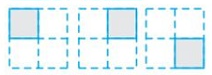(d)(i) – (d) Since 2×1/5=1/5+1/5
(ii) – (b) Since 2×1/2=1/2+1/2
(iii) – (a) Since 3×2/3=2/3+2/3+2/3
(iv) – (c) Since 3×1/4=1/4+1/4+1/4

2. Some pictures (a) to (c) are given below. Tell which of them show:
(i) 3×1/5 = 3/5
(ii) 2×1/3 = 2/3
(iii) 3×3/4 = 2.(1/4)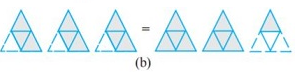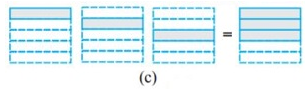(i) – (c) Since 3×1/5=1/5+1/5+1/5
(ii) – (a) Since 2×1/3=1/3+1/3
(iii) – (b) Since 3×3/4=3/4+3/4+3/4

3. Multiply and reduce to lowest form and convert into a mixed fraction:
(i) 7 × 3/5
(ii) 4 × 1/3
(iii) 2 × 6/7
(iv) 5 × 2/9
(v) 2/3 × 4
(vi) 5/2 × 6
(vii) 11 × 4/7
(viii) 20 × 4/5
(ix) 13 × 1/3
(x) 15 × 3/5

(i) 7× 3/5
= (7×3)/5
= 21/5
=4.(1/5)

(ii) 4× 1/3
= (4×1)/3
= 4/3
=1.(1/3)

(iii) 2× 6/7
= (2×6)/7
= 12/7
=1.(5/7)

(iv) 5× 2/9
= (5×2)/9
= 10/9
=1.(1/9)

(v) 2/3 × 4
= (2×4)/3
= 8/3
= 2.(2/3)

(vi) 5/2 × 6
= 5×3
= 15

(vii) 11× 4/7
= (11×4)/7
= 44/7
= 6.(2/7)

(viii) 20× 4/5
= 4×4
= 16

(ix) 13× 1/3
= (13×1)/3
= 13/3
=4.(1/3)

(x) 15× 3/5
= 3×3
= 9

(i) 2/3 of the triangles in box (a)
(ii) 3/5 of the squares in box (b)
(i) 1/2 of the circles in box (c)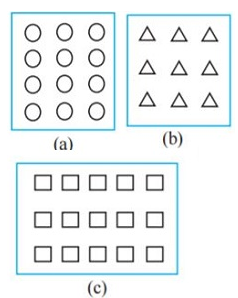(i) 2/3 of 9 triangles = 2/3 ×9 = 2×3 = 6 triangles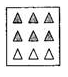(ii) 3/5 of 15 squares = 3/5 ×15 = 3 × 3 = 9 squares(iii) 1/2 of 12 circles = 1/2 ×12 = 6 circles5. Find:
(a) 1/2 of (i) 24 (ii) 46
(b) 2/3 of (i) 18 (ii) 27
(c) 3/4 of (i) 16 (ii) 36
(d) 4/5 of (i) 20 (ii) 35

(a)
(i) 1/2 of 24 = 12
(ii) 1/2 of 46 = 23

(b)
(i) 2/3 of 18 = 2/3×18 = 2 × 6 = 12
(ii) 2/3 of 27 = 2/3×27 = 2 × 9 = 18

(c)
(i) 3/4 of 16 = 3/4 × 16 = 3 × 4 = 12
(ii) 3/4 of 36 = 3/4 × 36 = 3 × 9 = 27

(d)
(i) 4/5 of 20 = 4/5 × 20 = 4 × 4 = 16
(ii) 4/5 of 35 = 4/5 × 35 = 4 × 7 = 28

6. Multiply and express as a mixed fraction:
(a) 3× 5.(1/5)
(b) 5× 6.(3/4)
(c) 7× 2.(1/4)
(d) 4× 6.(1/3)
(e) 3.(1/4) ×6
(f) 3.(2/5) ×8

(a) 3× 5.1/5
= 3× 26/5
= (3×26)/5
= 78/5
= 15.(3/5)

(b) 5× 6.(3/4)
= 5× 27/4
= (5×27)/4
= 135/4
= 33.(3/4)

(c) 7× 2.(1/4)
= 7× 9/4
= (7×9)/4
= 63/4
= 15.(3/4)

(d) 4×6.1/3
= 4× 19/3
= (4×19)/3
= 76/3
= 25.1/3

(e) 3.(1/4) ×6
= 13/4 ×6
= (13×3)/2
= 39/2
= 19.(1/2)

(f) 3.2/5×8
= 17/5 ×8
= (17×8)/5
= 136/5
= 27.(1/5)

7. Find:
(a) 1/2 of (i) 2.(3/4) (ii) 4.(2/9)
(b) 5/8 of (i) 3.(5/6) (ii) 9.(2/3)

(a)
(i) 1/2 of 2.(3/4) = 1/2×11/4 = 11/8 = 1.(3/8)
(ii) 1/2 of 4.(2/9) = 1/2 × 38/9 = 19/9 = 2.(1/9)

(b)
(i) 5/8 of 3.(5/6) = 5/8 × 23/6 = 115/48 = 2.(19/48)
(ii) 5/8 of 9.(2/3) = 5/8 × 29/3 = 145/24 = 6.(1/24)

8. Vidya and Pratap went for a picnic. Their mother gave them a water bottle that contained 5 litres of water. Vidya consumed 2/5 of the water. Pratap consumed the remaining water.
(i) How much water did Vidya drink?
(ii) What fraction of the total quantity of water did Pratap drink?

Total quantity of water in bottle = 5 litres
(i) Vidya consumed = 2/5 of 5 litres = 2/5×5 = 2 litres
Thus, Vidya drank 2 litres water from the bottle.
(ii) Pratap consumed = (1−2/5) part of bottle = 5−2/5=3/5 part of bottle
Pratap consumed 3/5 of 5 litres water = 3/5×5 = 3 litres
Thus, Pratap drank 3/5 part of the total quantity of water.

Page No. 41

Exercise 2.3

1. Find:
(i) 1/4 of
(a) 1/4
(b) 3/4
(c) 4/3
(ii)1/7 of
(a) 2/9
(b) 6/5
(c) 3/10

(i) (a) 1/4 of 1/4
= 1/4×1/4
=(1×1)/(4×4)
=1/16

(b) 1/4 of 3/4
= 1/4×3/4
=(1×3)/(4×4)
= 3/16

(c) 1/4 of 4/3
= 1/4×4/3
=(1×4)/(4×3)
=1/3

(ii) (a) 1/7 of 2/9
= 1/7×2/9
=(1×2)/(7×9)
=2/63

(b) 1/7 of 2/9
= 1/7×6/5
=(1×6)/(7×5)
=6/35

(c) 1/7 of 3/10
= 1/7×3/10
=(1×3)/(7×10)
=3/70

2. Multiply and reduce to lowest form (if possible):
(i) 2/3 × 2.(2/3)
(ii) 2/7 × 7/9
(iii) 3/8 × 6/4
(iv) 9/5 × 3/5
(v) 1/3 × 15/8
(vi) 11/2 × 3/10
(vii) 4/5 × 12/7

(i) 2/3 × 2.2/3
= 2/3 × 8/3
= (2×8)/(3×3)
= 16/9
= 1.(7/9)

(ii) 2/7 × 7/9
= (2×7)/(7×9)
= 2/9

(iii) 3/8 × 6/4
= (3×6)/(8×4)
= (3×3)/(8×2)
= 9/16

(iv) 9/5 × 3/5
= (9×3)/(5×5)
= 27/25
= 1.(2/25)

(v) 1/3 × 15/8
= (1×15)/(3×8)
= (1×5)/(1×8)
= 5/8

(vi) 11/2 × 3/10
=(11×3)/(2×10)
=33/20
=1.(3/20)

(vii) 4/5 × 12/7
=(4×12)/(5×7)
=48/35
=1.(13/35)

3. Multiply the following fractions:
(i) 2/5 × 5.(1/4)
(ii) 6.(2/5) × 7/9
(iii) 3/2 × 5.(1/3)
(iv) 5/6 × 2.(3/7)
(v) 3.(2/5) × 4/7
(vi) 2.(3/5) × 3
(vii) 3.(4/7) × 3/5

(i) 2/5 × 5.(1/4)
= 2/5 × 21/4
= (2×21)/(5×4)
= (1×21)/(5×2)
= 21/10
= 2.(1/10)

(ii) 6.(2/5) × 7/9
= 32/5 × 7/9
= (32×7)/(5×9)
= 224/45
= 4.(44/45)

(iii) 3/2×5.(1/3)
= 3/2 × 16/3
= 48/6
= 8

(iv) 5/6×2.(3/7)
= 5/6 × 17/7
= 85/42
= 2.(1/42)

(v) 3.(2/5) × 4/7
= 17/7 × 4/7
= 68/35
= 1.(33/35)

(vi) 2.(3/5) × 3
= 13/5 × 3/1
= (13×3)/(5×1)
= 39/5
= 7.(4/5)

(vii) 3.(4/7) × 3/5
= 25/7 × 3/5
= (5×3)/(7×1)
= 15/7
= 2.(1/7)

4. Which is greater:
(i) 2/7 of 3/4 or 3/5 of 5/8
(ii) 1/2 of 6/7 or 2/3 of 3/7

(i) 2/7 of 3/4 or 3/5 of 5/8
⇒ 2/7 × 3/4 or 3/5 × 5/8
⇒ 3/14 or 3/8
⇒ 3/14<3/8
Thus, 3/5 of 5/8 is greater.

(ii) 1/2 of 6/7 or 2/3 of 3/7
⇒ 1/2 × 6/7 or 2/3 × 3/7
⇒ 3/7 or 2/7
⇒ 3/7 > 2/7
Thus, 1/2 of 6/7 is greater.

5. Saili plants 4 saplings in a row in her garden. The distance between two adjacent saplings is 3/4 m. Find the distance between the first and the last sapling.

The distance between two adjacent saplings = 3/4 m
Saili planted 4 saplings in a row, then number of gap in saplingsTherefore, the distance between the first and the last saplings = 3×3/4 = 9/4m = 2.(1/4) m
Thus the distance between the first and the last saplings is 2.(1/4) m.

6. Lipika reads a book for 1.3/4 hours everyday. She reads the entire book in 6 days. How many hours in all were required by her to read the book?

Time taken by Lipika to read a book = 1.(3/4) hours.
She reads entire book in 6 days.
Now, total hours taken by her to read the entire book = 1.3/4×6 = 7/4×6=21/2=10.1/2 hours
Thus, 10 hours were required by her to read the book.

7. A car runs 16 km using 1 litre of petrol. How much distance will it cover using 2.3/4 litres of petrol?

In 1 litre of pertrol, car covers the distance = 16 km
In 2.3/4 litres of petrol, car covers the distance = 2.3/4 of 16 km = 11/4×16 = 44 km
Thus, car will cover 44 km distance.

8. (a) (i) Provide the number in the box ___ , such that 2/3× ___ = 10/30
(ii) The simplest form of the number obtained in ___ is _____.
(b) (i) Provide the number in the box ____ such that 3/5× ____ = 24/75
(ii) The simplest form of the number obtained in ___ is ____.

(a) (i) 2/3×5/10=10/30
(ii) The simplest form of 5/10 is 1/2
(ii) The simplest form of 8/15 is 8/15

Page No. 46

Exercise 2.4

1. Find:
(i) 12 ÷ 3/4
(ii) 14 ÷ 5/6
(iii) 8 ÷ 7/3
(iv) 4 ÷ 8/3
(v) 3 ÷ 2.(1/3)
(vi) 5 ÷ 3.(4/7)

(i) 12÷3/4 = 12 × 4/3 = 16
(ii) 14 ÷ 5/6 = 14 × 6/5 = 84/5 = 16.(4/5)
(iii) 8 ÷ 7/3 = 8× 3/7 = 24/7 = 3.(3/7)
(iv) 4÷ 8/3 = 4× 3/8 = 3/2 = 1.(1/2)
(v) 3÷ 2.(1/3) = 3÷ 7/3 = 3× 3/7 = 9/7 = 1.(2/7)
(vi) 5÷ 3.(4/7) = 5÷ 25/7 = 5× 7/25 = 7/5 = 1.(2/5)

2. Find the reciprocal of each of the following fractions. Classify the reciprocals as proper fraction, improper fractions and whole numbers.
(i) 3/7
(ii) 5/8
(iii) 9/7
(iv) 6/5
(v) 12/7
(vi) 1/8
(vii) 1/11

(i) Reciprocal of 3/7 = 7/3  → Improper fraction
(ii) Reciprocal of 5/8 = 8/5 → Improper fraction
(iii) Reciprocal of 9/7 = 7/9 → Proper fraction
(iv) Reciprocal of 6/5 = 5/6 →Proper fraction
(v)Reciprocal of 12/7 = 7/12 → Proper fraction
(vi) Reciprocal of 1/8 = 8 → Whole number
(vi) Reciprocal of 1/11 = 11 → Whole number

3. Find:
(i) 7/3 ÷2
(ii) 4/9 ÷5
(iii) 6/13 ÷7
(iv) 4.(1/3) ÷3
(v) 3.(1/2) ÷4
(vi) 4.(3/7) ÷7

(i) 7/3 ÷ 2 = 7/3 × ½ = (7×1)/(3×2) = 7/6 = 1.(1/6)
(ii) 4/9 ÷ 5 = 4/9 × 1/5 = (4×1)/(9×5) = 4/45
(iii) 6/13 ÷ 7 = 6/13 × 1/7 = (6×1)/(13×7) = 6/91
(iv) 4.(1/3) ÷ 3 = 13/3 ÷ 3 = 13/3 × 1/3 = 13/9 = 1.(4/9)
(v) 3.(1/2) ÷ 4 = 7/2 ÷ 4 = 7/2 × ¼ = 7/8
(vi) 4.(3/7) ÷ 7 = 31/7 ÷ 7 = 31/7 × 1/7 = 31/49

4. Find:
(i) 2/5 ÷ 1/2
(ii) 4/9 ÷ 2/3
(iii) 3/7 ÷ 8/7
(iv) 2.1/3 ÷ 3/5
(v) 3.1/2 ÷ 8/3
(vi) 2/5 ÷ 1.1/2
(vii) 3.1/5 ÷ 1.2/3
(viii) 2.1/5÷1.1/5

(i) 2/5 ÷ ½
= 2/5 × 2/1 = (2×2)/(5×1) =4/5

(ii) 4/9 ÷ 2/3
= 4/9 × 3/2 = 2/3

(iii) 3/7 ÷ 8/7
= 3/7 × 7/8 = 3/8

(iv) 2.(1/3) ÷ 3/5
= 7/3 ÷ 3/5 = 7/3 × 5/3 = 35/9 = 3.(8/9)

(v) 3.(1/2) ÷ 8/3
= 7/2 ÷ 8/3 = 7/2 × 3/8 = (7×3)/(2×8) = 21/16 = 1.(5/16)

(vi) 2/5 ÷ 1.(1/2)
= 2/5 ÷ 3/2 = 2/5 × 2/3 = (2×2)/(5×3) = 4/15

(vii) 3.(1/5) ÷ 1.(2/3)
= 16/5 ÷ 5/3 = 16/5 × 3/5 = (16×3)/(5×5) = 48/25 = 1.(23/25)

(viii) 2.(1/5) ÷ 1.(1/5)
= 11/5 ÷ 6/5 = 11/5 × 5/6 = 11/6 = 1.(5/6)

Page No. 47

Exercise 2.5

1. Which is greater:
(i) 0.5 or 0.05
(ii) 0.7 or 0.5
(iii) 7 or 0.7
(iv) 1.37 or 1.49
(v) 2.03 or 2.30
(vi) 0.8 or 0.88

(i) 0.50 > 0.05
(ii) 0.7 > 0.5
(iii) 7.0 > 0.7
(iv) 1.37 < 1.49
(v) 2.03 < 2.30
(vi) 0.80 < 0.88

2. Express as rupees using decimals:
(i) 7 paise
(ii) 7 rupees 7 paise
(iii) 77 rupees 77 paise
(iv) 50 paise
(v) 235 paise

∵ 100 paise = Re. 1
∴ 1 paisa = Re. 1/100
7 paise = Re. 7/100 = Re. 0.07
7 rupees 7 paise = Rs. 7 + Re. 7/100 = Rs. 7 + Re. 0.07 = Rs. 7.07
77 rupees 77 paise = Rs. 77 + Re. 77/100 = Rs. 77 + Re. 0.77 = Rs. 77.77
50 paise = Re. 50/100 = Re. 0.50
235 paise = Re. 235/100 = Rs. 2.35

3. (i) Express 5 cm in metre and kilometer.
(ii) Express 35 mm in cm, m and km.

(i) Express 5 cm in meter and kilometer.
∵ 100 cm = 1 meter
∴ 1 cm = 1/100 meter ⇒ 5 cm = 5/100 = 0.05 meter.
Now, ∵ 1000 meters = 1 kilometers
∴ 1 meter = 1/1000 kilometer
⇒ 0.05 meter = 0.05/1000 = 0.00005 kilometer

(ii) Express 35 mm in cm, m and km.
∵ 10 mm = 1 cm
∴ 1 mm = 1/10 cm ⇒ 35 mm = 35/10 = 3.5 cm
Now, ∵ 100 cm = 1 meter
∴ 1 cm = 1/100 meter ⇒3.5 cm = 3.5/100 = 0.035 meter
Again, ∵ 1000 meters = 1 kilometers
∴ 1 meter = 1/1000 kilometer
⇒ 0.035 meter = 0.035/1000 = 0.000035 kilometer

4. Express in kg.:
(i) 200 g
(ii) 3470 g
(iii)4 kg 8 g

1000 g = 1 kg ⇒ 1 g = 1/1000 kg

(i) 200 g = (200× 1/1000) kg = 0.2 kg
(ii) 3470 g = (3470× 1/1000) kg = 3.470 kg
(iii) 4 kg 8 g = 4 kg + (8× 1/1000) kg = 4 kg + 0.008 kg = 4.008 kg

5. Write the following decimal numbers in the expanded form:
(i) 20.03
(ii) 2.03
(iii) 200.03
(iv) 2.034

(i) 20.03 = 2×10 + 0×1 + 0×1/10 + 3×1/100
(ii) 2.03 = 2×1+0×1/10+3×1/100
(iii) 200.03 = 2×100+0×10+0×1+0×1/10+3×1/100
(iv) 2.034 = 2×1+0×1/10+3×1/100+4×1/1000

6. Write the place value of 2 in the following decimal numbers:
(i) 2.56
(ii) 21.37
(iii) 10.25
(iv) 9.42
(v) 63.352

(i) Place value of 2 in 2.56 = 2 × 1 = 2 ones
(ii) Place value of 2 in 21.37 = 2 × 10 = 2 tens
(iii) Place value of 2 in 10.25 = 2× 1/10 = 2 tenths
(iv) Place value of 2 in 9.42 = 2× 1/100 = 2 hundredth
(v) Place value of 2 in 63.352 = 2× 1/1000 = 2 thousandth

7. Dinesh went from place A to place B and from there to place C. A is 7.5 km from B and B is 12.7 km from C. Ayub went from place A to place D and from there to place C. D is 9.3 km from A and C is 11.8 km from D. Who travelled more and by how much?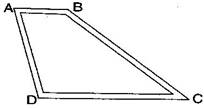Distance travelled by Dinesh when he went from place A to place B = 7.5 km and from place B to C = 12.7 km.Total distance covered by Dinesh = AB + BC
= 7.5 + 12.7 = 20.2 km
Total distance covered by Ayub = AD + DC
= 9.3 + 11.8 = 21.1 km
On comparing the total distance of Ayub and Dinesh,
21.1 km > 20.2 km
Therefore, Ayub covered more distance by 21.1 – 20.2 = 0.9 km = 900 m

8. Shyam bought 5 kg 300 g apples and 3 kg 250 g mangoes. Sarala bought 4 kg 800 g oranges and 4 kg 150 g bananas. Who bought more fruits?

Total weight of fruits bought by Shyam = 5 kg 300 g + 3 kg 250 g = 8 kg 550 g
Total weight of fruits bought by Sarala = 4 kg 800 g + 4 kg 150 g = 8 kg 950 g
On comparing the quantity of fruits,
8 kg 550 g < 8 kg 950 g
Therefore, Sarala bought more fruits.

9. How much less is 28 km than 42.6 km?

We have to find the difference of 42.6 km and 28 km.
42.6 – 28.0 = 14.6 km

Therefore 14.6 km less is 28 km than 42.6 km

Page No. 52

Exercise 2.6

1. Find:
(i) 0.2 × 6
(ii) 8 × 4.6
(iii) 2.71 × 5
(iv) 20.1 × 4
(v) 0.05 × 7
(vi) 211.02 × 4
(vii) 2 × 0.86

(i) 0.2 × 6 = 1.2
(ii) 8 × 4.6 = 36.8
(iii) 2.71 × 5 = 13.55
(iv) 20.1 × 4 = 80.4
(v) 0.05 × 7 = 0.35
(vi) 211.02 × 4 = 844.08
(vii) 2 × 0.86 = 1.72

2. Find the area of rectangle whose length is 5.7 cm and breadth is 3 cm ?

Given: Length of rectangle = 5.7 cm and Breadth of rectangle = 3 cm
Area of rectangle = Length × Breadth
= 5.7 × 3 = 17.1 cm2
Thus, the area of rectangle is 17.1 cm2

3. Find:
(i) 1.3 × 10
(ii) 36.8 × 10
(iii) 153.7 × 10
(iv) 168.07 × 10
(v) 31.1 × 100
(vi) 156.1 × 100
(vii) 3.62 × 100
(viii) 3.07 × 100
(ix) 0.5 × 10
(x) 0.08 × 10
(xi) 0.9 × 100
(xii) 0.03 × 1000

(i) 1.3 × 10 = 13.0
(ii) 36.8 × 10 = 368.0
(iii) 153.7 × 10 = 1537.0
(iv) 168.07 × 10 = 1680.7
(v) 31.1 × 100 = 3110.0
(vi) 156.1 × 100 = 15610.0
(vii) 3.62 × 100 = 362.0
(viii) 43.07 × 100 = 4307.0
(ix) 0.5 × 10 = 5.0
(x) 0.08 × 10 = 0.80
(xi) 0.9 × 100 = 90.0
(xii) 0.03 × 1000 = 30.0

4. A two-wheeler covers a distance of 55.3 km in one litre of petrol. How much distance will it cover in 10 litres of petrol?

∵ In one litre, a two-wheeler covers a distance = 55.3 km
∴ In 10 litrs, a two- wheeler covers a distance = 55.3 × 10 = 553.0 km
Thus, 553 km distance will be covered by it in 10 litres of petrol.

5. Find:
(i) 2.5 × 0.3
(ii) 0.1 × 51.7
(iii) 0.2 × 316.8
(iv) 1.3 × 3.1
(v) 0.5 × 0.05
(vi) 11.2 × 0.15
(vii) 1.07 × 0.02
(viii) 10.05 × 1.05
(ix) 101.01 × 0.01
(x) 100.01 × 1.1

(i) 2.5 × 0.3 = 0.75
(ii) 0.1 × 51.7 = 5.17
(iii) 0.2 × 316.8 = 63.36
(iv) 1.3 × 3.1 = 4.03
(v) 0.5 × 0.05 = 0.025
(vi) 11.2 × 0.15 = 1.680
(vii) 1.07 × 0.02 = 0.0214
(viii) 10.05 × 1.05 = 10.5525
(ix) 101.01 × 0.01 = 1.0101
(x) 100.01 × 1.1 = 110.11

Page No. 55

Exercise 2.7

1. Find:
(i) 0.4 ÷ 2
(ii) 0.35 ÷ 5
(iii) 2.48 ÷ 4
(iv) 65.4 ÷ 6
(v) 651.2 ÷ 4
(v) 14.49 ÷ 7
(vii) 3.96 ÷ 4
(viii) 0.80 ÷ 5

(i) 0.4 ÷ 2
= 4/10 × 1/ 2= 2/10 = 0.2

(ii) 0.35 ÷ 5
= 35/100 × 1/5 = 7/100 = 0.07

(iii)2.48 ÷ 4
= 248/100 × 1/4 = 62/100 = 0.62

(iv) 65.4 ÷ 6
= 654/10 × 1/6 = 109/10 = 10.9

(v) 651.2 ÷ 4
= 6512/10 × 1/4 = 1628/10 = 162.8

(vi) 14.49 ÷ 7
= 1449/100 × 1/7 = 207/100 = 2.07

(vii) 3.96 ÷ 4
= 396/100 × 1/4 = 99/100 = 0.99

(viii) 0.80 ÷ 5
= 80/100 × 1/5 = 16/100 = 0.16

2. Find:
(i) 4.8 ÷ 10
(ii) 52.5 ÷ 10
(iii) 0.7 ÷ 10
(iv) 33.1 ÷ 10
(v) 272.23 ÷ 10
(vi) 0.56 ÷ 10
(vii) 3.97 ÷ 10

(i) 4.8 ÷ 10
= 4.8/10 = 0.48

(ii) 52.5 ÷ 10
= 52.5/10 = 5.25

(iii) 0.7 ÷ 10
= 0.7/10 = 0.07

(iv) 33.1 ÷ 10
= 33.1/10 = 3.31

(v) 272.23 ÷ 10
= 272.23/10 = 27.223

(vi) 0.56 ÷ 10
= 0.56/10 = 0.056

(vii) 3.97 ÷ 10
= 3.97/10 = 0.397

3. Find:
(i) 2.7 ÷ 100
(ii) 0.3 ÷ 100
(iii) 0.78 ÷ 100
(iv) 432.6 ÷ 100
(v) 23.6 ÷ 100
(vi) 98.53 ÷ 100

(i) 2.7 ÷ 100
= 27/10 × 1/100 = 27/1000 = 0.027

(ii) 0.3 ÷ 100
= 3/10 × 1/100 =3/1000 = 0.003

(iii) 0.78 ÷ 100
= 78/100 × 1/100 = 78/10000 = 0.0078

(iv) 432.6 ÷ 100
= 4326/10 × 1/100 = 4326/1000 = 4.326

(v) 23.6 ÷ 100
= 236/10 × 1/100 = 236/1000 = 0.236

(vi) 98.53 ÷ 100
= 9853/100 × 1/100 = 9853/10000 = 0.9853

4. Find:
(i) 7.9 ÷ 1000
(ii) 26.3 ÷ 1000
(iii) 38.53 ÷ 1000
(iv) 128.9 ÷ 1000
(v) 0.5 ÷ 1000

(i) 7.9 ÷ 1000
= 79/10 × 1/1000 = 79/10000 = 0.0079

(ii) 26.3 ÷ 1000
= 263/10 × 1/1000 = 263/10000 = 0.0263

(iii) 38.53 ÷ 1000
= 3853/100 × 1/1000 = 3853/100000 = 0.03853

(iv) 128.9 ÷ 1000
= 1289/10 × 1/1000 = 1289/10000 = 0.1289

(v) 0.5 ÷ 1000
= 5/10 × 1/1000 = 5/10000 = 0.0005

5. Find:
(i) 7 ÷ 3.5
(ii) 36 ÷ 0.2
(iii) 3.25 ÷ 0.5
(iv) 30.94 ÷ 0.7
(v) 0.5 ÷ 0.25
(vi) 7.75 ÷ 0.25
(vii) 76.5 ÷ 0.15
(viii) 37.8 ÷ 1.4
(ix) 2.73 ÷ 1.3

(i) 7 ÷ 3.5
= 7 ÷ 35/10 = 7 × 10/35 = 10/5 = 2

(ii) 36 ÷ 0.2
= 36 ÷ 2/10 = 36 × 10/2 = 18 × 10 = 180

(iii) 3.25 ÷ 0.5
= 325/100 ÷ 5/10 = 325/100 × 10/5 = 65/10 = 6.5

(iv) 30.94 ÷ 0.7
= 3094/100 ÷ 7/10 = 3094/100 × 10/7 = 442/10 = 44.2

(v) 0.5 ÷ 0.25
= 5/10 ÷ 25/100 = 5/10 × 100/25 = 10/5 = 2

(vi) 7.75 ÷ 0.25
= 775/100 ÷ 25/100 = 775/100 × 100/25 = 31

(vii) 76.5 ÷ 0.15
= 765/10 ÷ 15/100 = 765/10 × 100/15 = 51×10 = 510

(viii) 37.8 ÷ 1.4
= 378/10 ÷ 14/10 = 378/10 × 10/14 = 27

(ix) 2.73 ÷ 1.3
= 273/100 ÷ 13/10 = 273/100 × 10/13 = 21/10 = 2.1

6. A vehicle covers a distance of 43.2 km in 2.4 litres of petrol. How much distance will it cover in one litre petrol?

∵ In 2.4 litres of petrol, distance covered by the vehicle = 43.2 km
∴ In 1 litre of petrol, distance covered by the vehicle = 43.2 ÷ 2.4
= 432/10 ÷ 24/10 = 432/10 × 10/24
= 18 km
Thus, it covered 18 km distance in one litre of petrol.

Go Back To NCERT Solutions for Class 7 Maths

## NCERT Solutions for Class 7 Maths Chapter 2 Fractions and Decimals

NCERT Solutions for Chapter 2 Fractions and Decimals will guide student in a better way and help in revising the chapter properly. Fractions are types of integers and are used to represent a part of the whole. They are represented in the format of “p/q” where the “p” part is called the Numerator and the “q” part is called the Denominator. P/q means ‘p’ parts out of ‘q’ number of total parts. Decimal numbers are fractions with the divisor as 10 or multiples of 10.

• The product of two proper fractions is less than each of the fractions that are multiplied.

• The product of a proper and an improper fraction is less than the improper fraction and greater than the proper fraction.

• The product of two improper fractions is greater than the two fractions.

• To multiply a decimal number by 10, 100 or 1000, we move the decimal point in the number to the right by as many places as there are zeros over 1.

You will also find exercisewise Class 7 Maths NCERT Solutions below through which one can find specific question that you're looking for. It can be used to enrich knowledge and make lessons for learners more exciting.

These NCERT Solutions will help you in analyzing the problems and answering it with precision and the right concepts. It will encourage students to learn new topics and checking your own answers.

### NCERT Solutions for Class 7 Maths Chapters:

 Chapter 1 Integers Chapter 3 Data Handling Chapter 4 Simple Equations Chapter 5 Lines and Angles Chapter 6 The Triangle and its Properties Chapter 7 Congruence of Triangles Chapter 8 Comparing Quantities Chapter 9 Rational Numbers Chapter 10 Practical Geometry Chapter 11 Perimeter and Area Chapter 12 Algebraic Expressions Chapter 13 Exponents and Powers Chapter 14 Symmetry Chapter 15 Visualising Solid Shapes

FAQ on Chapter 2 Fractions and Decimals

#### How many exercises are there in Chapter 2 Fractions and Decimals Class 7 Maths NCERT Solutions?

Chapter 2 Class 7 Maths consist of 7 exercises will give good experience and provide opportunities to learn new things. Students can develop their understanding of the chapter and obtain maximum marks in the exams.

#### Multiply 21/5 and 15/4.

Converting the mixed fraction to normal we get 11/5.
Now,  11/5 × 15/4
= (11 × 15)/(5 × 4)
Canceling common terms,
=( 11×  5 × 3)/ (5× 4)
= 33/4.

#### Obtain the result if (2/3) is added to (4/9) and is multiplied by 3.

3 × (2/3 + 4/9)
= 3 × (6/9 + 4/9)
= 3 × ( 10/9)
= 30/9
= 10/3.3333333333333333.

#### Divide 7 by 23/4.

Now converting the whole number to a fraction and converting mixed fraction to regular fraction
= 7/1 and 11/4
taking reciprocal of the second fraction and multiplying,
7/1 × 4/11
= 28/11
= 26/11.